特III型晓级驱逐舰二番舰 响 （栀） IJN Hibiki 85px 编号 NO.160 类型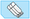驱逐 稀有度 未实装未实装 阵营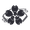重樱 建造时间 未实装 普通掉落点 活动掉落点 营养价值 待补充 退役收益 强化所需经验 炮击 *=0 雷击 *=0 航空 *=0 装填 *=0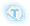+

0+
-
Lv.120+

canvas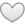耐久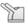防空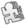机动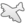航空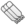雷击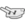炮击

 初始装备 装备说明 槽位/装备类型/武器效率初始/满破/武器数满破/预装填数满破 槽 装备类型 效率 武器数 预装填数 1 驱逐炮 / 2 水面舰艇鱼雷 / 3 防空炮 / 4 设备 - - - 5 设备 - - - 注 水面舰艇鱼雷底座+1与航母机库+1并不增加武器数 1 - 2 - 3 -
 技能；
 前排弹幕或后排专属弹幕 请上传文件『响弹幕.gif』，文件大小不超过2M，上传完毕后，编辑刷新。
 立绘 通常换装改造誓约 请上传文件『响立绘.jpg』，文件大小不超过2M。 请上传文件『响换装.jpg』，文件大小不超过2M。 请上传文件『响改造.jpg』，文件大小不超过2M。 请上传文件『响誓约.jpg』，文件大小不超过2M。 角色信息 实装 身份 性格 关键词 持有物 发色 瞳色 萌点 CV 画师 + 微博 - 推特 - PIXIV
 评价 备注

登陆界面 碧蓝航线！

## 其它舰娘

驱逐 • 卡辛改 • 唐斯改 • 格里德利 • 克雷文 • 麦考尔 • 莫里 • 弗莱彻 • 查尔斯·奥斯本 • 撒切尔 • 奥利克 • 富特 • 斯彭斯 • 拉德福特 • 杰金斯 • 尼古拉斯改 • 布什 • 黑泽伍德 • 贝奇 • 金伯利 • 马拉尼 • 斯坦利 • 斯莫利 • 本森 • 拉菲改 • 贝利改 • 霍比 • 科尔克 • 西姆斯改 • 哈曼改 • 埃尔德里奇 • 杜威 • 艾尔温 • 亚特兰大 • 朱诺 • 圣地亚哥改 • 小圣地亚哥 • 圣胡安 • 奥马哈 • 罗利 • 里士满 • 孟菲斯 • 康克德 • 布鲁克林 • 菲尼克斯 • 海伦娜 • 火奴鲁鲁 • 圣路易斯 • 小海伦娜 • 克利夫兰 • 哥伦比亚 • 蒙彼利埃 • 丹佛 • 小克利夫兰 • 伯明翰 • 克利夫兰(μ兵装) • 西雅图 • 彭萨科拉 • 盐湖城 • 北安普敦 • 芝加哥 • 休斯敦 • 波特兰改 • 印第安纳波利斯 • 威奇塔 • 阿斯托利亚 • 昆西 • 文森斯 • 明尼阿波利斯 • 巴尔的摩 • 内华达改 • 俄克拉荷马改 • 宾夕法尼亚 • 亚利桑那 • 田纳西 • 加利福尼亚 • 科罗拉多 • 马里兰 • 西弗吉尼亚 • 北卡罗来纳 • 华盛顿 • 南达科他 • 马萨诸塞 • 阿拉巴马 • 佐治亚 • 长岛改 • 博格改 • 兰利改 • 突击者改 • 独立 • 巴丹 • 列克星敦 • 萨拉托加改 • 约克城 • 企业 • 大黄蜂 • 胡蜂 • 埃塞克斯 • 邦克山 • 香格里拉 • 鲦鱼 • 大青花鱼 • 棘鳍 • 女灶神
驱逐 • 女将 • 阿卡司塔改 • 热心改 • 小猎兔犬 • 大斗犬 • 彗星改 • 新月改 • 小天鹅改 • 狐提改 • 命运女神改 • 格伦维尔 • 萤火虫 • 勇敢 • 猎人 • 吸血鬼 • 标枪改 • 天后 • 丘比特 • 泽西 • 无敌 • 火枪手 • 回声 • 利安得改 • 阿基里斯改 • 阿贾克斯改 • 南安普顿 • 谢菲尔德 • 格拉斯哥 • 纽卡斯尔改 • 谢菲尔德(μ兵装) • 阿瑞托莎 • 加拉蒂亚 • 欧若拉 • 斐济 • 牙买加 • 爱丁堡 • 贝尔法斯特 • 小贝法 • 天狼星 • 黑太子 • 库拉索改 • 杓鹬改 • 确捷 • 海王星 • 伦敦改 • 什罗普郡 • 苏塞克斯 • 肯特 • 萨福克改 • 诺福克 • 多塞特郡 • 约克改 • 埃克塞特改 • 声望 • 反击 • 胡德 • 伊丽莎白女王 • 厌战改 • 纳尔逊 • 罗德尼 • 英王乔治五世 • 威尔士亲王 • 约克公爵 • 君主 • 竞技神改 • 独角兽 • 半人马 • 追赶者 • 皇家方舟 • 光辉 • 胜利 • 可畏 • 光荣 • 黑暗界 • 恐怖 • 阿贝克隆比
驱逐 • 吹雪 • 绫波改 • 晓 • 雷 • 电 • 白露 • 夕立 • 时雨改 • 江风 • 雪风 • 阳炎改 • 不知火改 • 野分 • 黑潮 • 亲潮 • 浦风 • 矶风 • 滨风改 • 谷风改 • 初春 • 若叶 • 初霜 • 有明 • 夕暮改 • 神风改 • 松风改 • 旗风 • 睦月改 • 如月改 • 卯月 • 水无月 • 文月 • 长月 • 三日月 • 新月 • 春月 • 宵月 • 朝潮 • 大潮 • 满潮 • 荒潮 • 清波 • 卷波 • 北风 • 夕张改 • 长良 • 五十铃 • 阿武隈改 • 最上改 • 三隈 • 川内改 • 神通改 • 那珂 • 阿贺野 • 古鹰改 • 加古改 • 青叶 • 衣笠 • 妙高 • 那智 • 足柄 • 高雄 • 爱宕 • 摩耶 • 鸟海 • 铃谷 • 伊吹 • 金刚 • 比叡 • 榛名 • 雾岛 • 小比叡 • 天城 • 扶桑改 • 山城改 • 伊势改 • 日向改 • 长门 • 陆奥 • 加贺BB • 三笠 • 出云 • 飞鹰 • 隼鹰 • 祥凤改 • 凤翔 • 龙骧 • 赤城 • 加贺 • 小赤城 • 赤城(μ兵装) • 苍龙改 • 飞龙改 • 翔鹤 • 瑞鹤 • 大凤 • 伊19 • 伊26 • 伊25 • 伊58 • 伊56 • 伊168 • 明石 • 伊13 • 吾妻
驱逐 • Z1改 • Z2 • Z18 • Z19 • Z20 • Z21 • Z23改 • Z25 • Z35 • Z36 • Z46 • 柯尼斯堡 • 卡尔斯鲁厄改 • 科隆改 • 莱比锡改 • 希佩尔海军上将 • 欧根亲王 • 希佩尔海军上将(μ兵装) • 德意志 • 斯佩伯爵海军上将 • 罗恩 • 沙恩霍斯特 • 格奈森瑙 • 俾斯麦 • 提尔比茨 • 腓特烈大帝 • 齐柏林伯爵 • 小齐柏林 • U-81 • U-557 • U-556 • U-47 • U-73 • U-101 • U-522 • U-110
驱逐 • 鞍山 • 抚顺 • 长春 • 太原 • 逸仙 • 宁海改 • 平海改
驱逐 • 龙骑兵 • 特伦托 • 扎拉 • 利托里奥 • 加富尔伯爵 • 朱利奥·凯撒
轻巡 • 阿芙乐尔
驱逐 • 凯旋 • 福尔班改 • 鲁莽 • 倔强 • 埃米尔·贝尔汀改 • 路易九世 • 絮库夫
驱逐 • 勒马尔改 • 恶毒 • 敦刻尔克 • 让·巴尔 • 加斯科涅(μ兵装) • 加斯科涅
驱逐 • 泛用型布里 • 试作型布里MKII
驱逐 • 布兰 • 群白之心 • 涅普顿 • 绀紫之心 • 诺瓦露 • 圣黑之心 • 贝露 • 翡绿之心
驱逐 • 22 • 33
驱逐 • 猫音 • 露露缇耶 • 久远 • 乌璐露 • 萨拉娜 • 芙米露露
驱逐 • 绊爱 • 绊爱·Elegant • 绊爱·SuperGamer • 绊爱·Anniversary